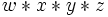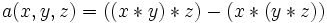# Associativity pentagon

Jump to: navigation, search

## Definition

The associativity pentagon is a pentagon whose five vertices are the five different ways of parenthesizing the product$w * x * y * z$, with an edge between two parenthesizations if we can move from one to the other by a single application of the associativity law. The five parenthesizations are, in cyclic order:

•$((w * x) * y) * z$.
•$(w * x) * (y * z)$.
•$w * ((x * (y * z))$.
•$w * ((x * y) * z)$.
•$(w * (x * y)) * z$.

## Generalizations

The associativity pentagon is the case$n = 4$ of the associahedron$K_n$, which is a convex polytope whose vertices are all the possible different parenthesizations of a product string of length$n$.

## Importance

### Proving results about situations where associativity is true for only some elements

The associativity pentagon tells us that given any two different parenthesizations of an expression, there are two routes from one to the other by iterated application of the associativity law. In situations where we have weak forms of associativity, we may use one route in order to prove the validity of the other route. Examples are:

### The associator identity

Further information: associator identity

Suppose$R$ is a non-associative ring. We can define the associator$a:R^3 \to R$:$\! a(x,y,z) = ((x * y) * z) - (x * (y * z))$

Then,$a$ satisfies an identity with five terms and four variables (universally quantified) that essentially corresponds to the process of going around the associativity pentagon. This identity is called the associator identity, and is given by:$\! w * a(x,y,z) + a(w,x,y) * z = a(w*x,y,z) - a(w,x*y,z) + a(w,x,y*z)$

### Putting the pentagon axiom on natural isomorphisms meant to mimic associativity

In algebra, associativity means a strict equality between two different parenthesizations. On the other hand, in the study of categories, where the set is replaced by a category and the binary operation by a monoidal operation that is functorial in both variables, strict associativity in the sense of identity does not hold. Rather, we postulate the existence of natural associativity isomorphisms for those operations that we want to think of as associative. In other words, we define, for every triple$a,b,c$ of objects, a natural isomorphism from$a * (b * c)$ to$(a * b) * c$.

We want this isomorphism to work as follows: given any two routes from one parenthesization to the other, the composite of the natural isomorphisms along the way on both routes must be the same. In particular, we want the pentagon axiom: applying the natural isomorphisms by moving five steps along the pentagon should give the identity map. It turns out that the pentagon axiom, along with the naturality assumption, is sufficient to guarantee the equivalence of any two routes between two parenthesizations. In other words, the associativity pentagon generates all relations between the associative laws.